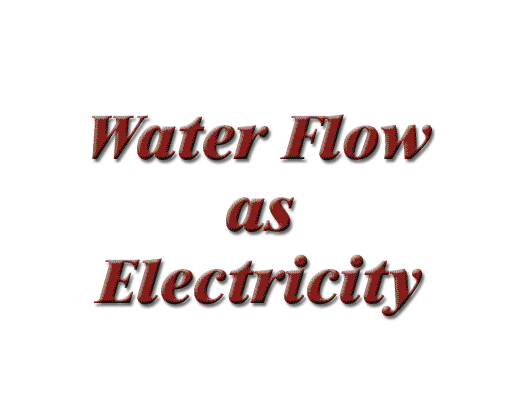# Ohm’s Law

Water flow is often used to describe electricity. In the water analogy, water is the medium to transfer force. In an electrical circuit, the medium is the “free” electrons of conductive metals like copper or aluminum. Water transmits force through the water in a pipe; in an electrical circuit, the force is transmitted over the electrons of a conductor. In our moving picture, we use water to turn a waterwheel. The weight of the water in the tower exerts pressure on the outflow pipe. As the water flows out and under the paddles of the waterwheel, most of the water’s kinetic energy (objects in motion) has been converted into rotational force (torque) at the axle of our illustrative contraption. Pressure is said to be dropped across the load. To maintain pressure at the source, new energy must be added—somebody has to scoop up the water, climb the tower and keep the water level constant. In an electrical system, it is the utility that must maintain this pressure, known as voltage.
The force exerted on the axle depends on three things: The pressure (volts) in the pipe, the gallons per minute of water flow (amps), and the restrictive effect of the pipe and valve diameter (ohms). There is a simple formula to express this relationship of pressure, flow rate, and resistance. The formula is called Ohm’s law after the German scientist George Simon Ohm who first described it. It reads:

E = IR, or in lay terms,
Volts = Amps x Ohms

E = electromotive force = V = voltage
I = intensity = A = current (measured in amperes)
R = resistance in ohms

###### next page >